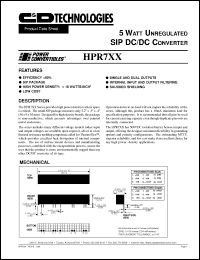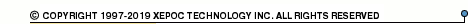More than4 307 195 components listedHPR7 series datasheets. Manufacturer: CANDD.

 HPR700 5 Watt unregulated DC/DC converter. Nom.input voltage 5VDC, rated output voltage 5VDC, rated output current 1000mA. in 20-pin SIP package. Operational temperature range from -25°C to 70°C. Datasheet*) HPR700H 5 Watt unregulated DC/DC converter. Nom.input voltage 5VDC, rated output voltage 5VDC, rated output current 1000mA. in 20-pin SIP package. Operational temperature range from -25°C to 70°C. Datasheet*) HPR703 5 Watt unregulated DC/DC converter. Nom.input voltage 5VDC, rated output voltage +-5VDC, rated output current +-500mA. in 20-pin SIP package. Operational temperature range from -25°C to 70°C. Datasheet*) HPR703H 5 Watt unregulated DC/DC converter. Nom.input voltage 5VDC, rated output voltage +-5VDC, rated output current +-500mA. in 20-pin SIP package. Operational temperature range from -25°C to 70°C. Datasheet*) HPR704 5 Watt unregulated DC/DC converter. Nom.input voltage 5VDC, rated output voltage +-12VDC, rated output current +-208mA. in 20-pin SIP package. Operational temperature range from -25°C to 70°C. Datasheet*) HPR704H 5 Watt unregulated DC/DC converter. Nom.input voltage 5VDC, rated output voltage +-12VDC, rated output current +-208mA. in 20-pin SIP package. Operational temperature range from -25°C to 70°C. Datasheet*) HPR705 5 Watt unregulated DC/DC converter. Nom.input voltage 5VDC, rated output voltage +-15VDC, rated output current +-167mA. in 20-pin SIP package. Operational temperature range from -25°C to 70°C. Datasheet*) HPR705H 5 Watt unregulated DC/DC converter. Nom.input voltage 5VDC, rated output voltage +-15VDC, rated output current +-167mA. in 20-pin SIP package. Operational temperature range from -25°C to 70°C. Datasheet*) HPR706 5 Watt unregulated DC/DC converter. Nom.input voltage 12VDC, rated output voltage 5VDC, rated output current 1000mA. in 20-pin SIP package. Operational temperature range from -25°C to 70°C. Datasheet*) HPR706H 5 Watt unregulated DC/DC converter. Nom.input voltage 12VDC, rated output voltage 5VDC, rated output current 1000mA. in 20-pin SIP package. Operational temperature range from -25°C to 70°C. Datasheet*) HPR710 5 Watt unregulated DC/DC converter. Nom.input voltage 12VDC, rated output voltage +-12VDC, rated output current +-208mA. in 20-pin SIP package. Operational temperature range from -25°C to 70°C. Datasheet*) HPR710H 5 Watt unregulated DC/DC converter. Nom.input voltage 12VDC, rated output voltage +-12VDC, rated output current +-208mA. in 20-pin SIP package. Operational temperature range from -25°C to 70°C. Datasheet*) HPR711 5 Watt unregulated DC/DC converter. Nom.input voltage 12VDC, rated output voltage +-15VDC, rated output current +-167mA. in 20-pin SIP package. Operational temperature range from -25°C to 70°C. Datasheet*) HPR711H 5 Watt unregulated DC/DC converter. Nom.input voltage 12VDC, rated output voltage +-15VDC, rated output current +-167mA. in 20-pin SIP package. Operational temperature range from -25°C to 70°C. Datasheet*) HPR712 5 Watt unregulated DC/DC converter. Nom.input voltage 15VDC, rated output voltage 5VDC, rated output current 1000mA. in 20-pin SIP package. Operational temperature range from -25°C to 70°C. Datasheet*) HPR712H 5 Watt unregulated DC/DC converter. Nom.input voltage 15VDC, rated output voltage 5VDC, rated output current 1000mA. in 20-pin SIP package. Operational temperature range from -25°C to 70°C. Datasheet*) HPR717 5 Watt unregulated DC/DC converter. Nom.input voltage 15VDC, rated output voltage +-15VDC, rated output current +-167mA. in 20-pin SIP package. Operational temperature range from -25°C to 70°C. Datasheet*) HPR717H 5 Watt unregulated DC/DC converter. Nom.input voltage 15VDC, rated output voltage +-15VDC, rated output current +-167mA. in 20-pin SIP package. Operational temperature range from -25°C to 70°C. Datasheet*) HPR7XX 5 WATT UNREGULATED SIP DC/DC CONVERTER Datasheet*)